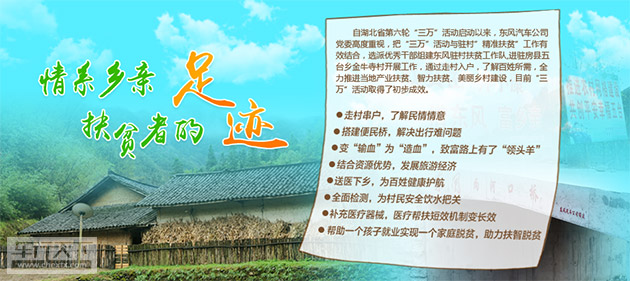## 东风干部“转型”搞养殖 卖山货有点忙

2017年06月14日 10:43来源：www.chextx.com超过：8040次关注

作为央企，东风公司在主动参与精准扶贫工作中，充分发挥央企的责任担当和示范引领作用，打造了一系列特色扶贫项目，赢得了湖北房县、广西马山县、新疆柯坪县、西藏贡觉等东风精准扶贫点百姓们们的点赞。今天，我们就来一起聊聊东风驻湖北房县五台乡金牛寺村的扶贫事儿。金牛寺村位于十堰房县五台乡东南部，因地理位置偏僻，基础设施落后，有效耕地缺乏，村集体经济为零，成为省级重点贫困村。全村306户、978人，其中贫困户达到164户、405人，年人均收入远落后于房县平均水平。东风公司按照中央和湖北省委精准扶贫要求，积极履行企业社会责任，于2015年10月底选派2名优秀干部组建东风扶贫工作队进驻金牛寺村，全力参与房县精准扶贫。精准扶贫是一场战役。2016年，是东风公司铁路运输处边焕新和十堰管理部张文涛哥俩组成东风公司扶贫工作队，入驻扶贫的第一个完整年。这一年，他俩谨记责任，情系村民，坚持以改善民生为重点，实施基础设施建设扶贫、产业扶贫、公共服务扶贫、扶智脱贫、社会关爱扶贫等多项口碑工程，帮扶工作初见成效。全年累计投入115.6万元，带动投资251万元，金牛寺村村集体收入实现了从0到5.02万元的“大突破”。

将五台乡打造成一个绿色农副产品供应基地，是东风扶贫工作队在对五台乡进行近两年扶贫之后定下的扶贫战略。今年，是“哥俩”扶贫工作组在这个村工作的第三个年头，除了看到了乡亲们脱贫致富的笑脸之外，在这个偏远的乡村又响起了产业扶贫的掌声。到底怎么回事呢？这跟今年“跑跑鸡”、黑山羊项目热销不愁卖有关。土鸡是东风扶贫工作队与万德福超市的第一个合作产品。东风扶贫工作队先后与东风公司人事（干部）部、湖北某大型餐饮企业、十堰连锁超市万德福等洽谈合作，力求借势东风为五台乡农副产品打通销路。端午节前，290只房县五台乡土鸡上架东风万得福超市，在万德福电商平台福E购和超市货柜同步销售。这种放养山野的土鸡被戏称为“跑跑鸡”，生长周期在一年左右，口味媲美野山鸡，上架三天内即售空，市场好评一片。黑山羊又是东风扶贫工作队为村民发家致富确定的第一个市场产品。如今，金牛寺村黑山羊养殖基地一期顺利建成，羔羊已经入住“别墅”，二期工程正在准备过程中，预计未来将形成1500只的养殖能力和规模。一个公司、一个基地、一个合作社，带动一批农户养殖种植，帮助一批农户就业，养殖种植一体化的循环发展模式正在五台乡逐步建立。“打算先养20只黑山羊试试水，至少有15元一斤的保护价，比现在家里养的羊价格高。”周平就是金牛寺村第一个为养殖黑山羊修建标准化羊栏的村民。 “村民抗风险能力低，每一笔投入都要咬咬牙，总收入少一点就会影响生活，所以一定要让每个响应扶贫产业的家庭赚到钱。”在边焕新看来，“卖出去、卖得好”远比“养得多”重要，所以他一开始就想方设法引入养殖公司，为村民养殖装上“保险丝”。除了山羊之外，土鸡、黄牛、山中蔬果也要尽快找到稳定的市场。只要有了销路，市场有保障，产业扶贫才能真正出实效出长效。东风扶贫工作队边焕新与张文涛深知自己在这里的责任。夏去秋来，我作为东风公司驻金牛寺村扶贫工作组成员在这里已经第三个年头了，我了解了这里的山山水水，爱上了这里淳朴的百姓们。在这里的每一天，做的每一件事都要与“责、情、法”三个字关联起来。“责”是时刻把东风的责任记心上，要在国家建设小康社会的扶贫攻坚战中留下东风公司值得称道的印迹。“情”是情系百姓，情系百姓增收，让百姓感受东风的温暖。“法”是用正确的方法工作，摆正位置，与所在地政府协同工作，发挥东风大家庭的优势，尽心尽力、尽善尽美做好精准扶贫任务。从城市到农村，从机关到山野，不是一种个人生活体验，而是帮助百姓全体脱贫致富的重任。这三年深有体会：一是懂政策，察民情，才能开展扶贫工作；二是说农村话、办农村事，才能融入工作新环境；三是抓住重点，掌控关键，是扶贫工作落地显效的关键。当前，我们只有以产业脱贫为核心，把“公司+合作社+农户”特色鲜明的扶贫模式运行好，让每一个农户走向产业致富的小康之路。

#### 相关文章

0-500 字已有评论 0条 查看评论>>

### 热门标签

﻿
• 快速找车
• 选择品牌
• 选择品牌
• A  奥迪
• A  阿斯顿·马丁
• A  阿尔法·罗密欧
• B  宝沃
• B  布加迪
• B  巴博斯
• B  保时捷
• B  宾利
• B  奔驰
• B  宝马
• B  本田
• B  别克
• B  标致
• B  比亚迪
• B  宝骏
• B  北汽制造
• B  北汽新能源
• B  北汽幻速
• B  北汽威旺
• B  北京汽车
• B  奔腾
• B  北汽绅宝
• C  长安
• C  长安商用
• C  长城
• C  昌河
• D  大众
• D  道奇
• D  DS
• D  东南
• D  东风风神
• D  东风风行
• D  东风小康
• D  东风风度
• D  东风
• F  福特
• F  丰田
• F  菲亚特
• F  法拉利
• F  福田
• F  福迪
• F  福汽启腾
• G  观致
• G  广汽传祺
• G  广汽吉奥
• G  GMC
• H  红旗
• H  汉腾汽车
• H  哈弗
• H  哈飞
• H  海格
• H  海马
• H  华颂
• H  黄海
• H  华泰
• H  恒天
• J  吉利汽车
• J  捷豹
• J  Jeep
• J  江淮
• J  江铃
• J  金杯
• J  九龙
• J  金旅
• K  凯翼
• K  凯迪拉克
• K  克莱斯勒
• K  科尼塞克
• K  卡威
• K  开瑞
• L  路虎
• L  林肯
• L  劳斯莱斯
• L  兰博基尼
• L  雷克萨斯
• L  铃木
• L  雷诺
• L  理念
• L  力帆
• L  莲花汽车
• L  猎豹
• L  路特斯
• L  陆风
• M  马自达
• M  MG
• M  MINI
• M  玛莎拉蒂
• M  摩根
• M  迈凯轮
• N  纳智捷
• O  欧宝
• O  讴歌
• O  欧朗
• Q  奇瑞
• Q  起亚
• Q  启辰
• R  日产
• R  荣威
• R  瑞麒
• S  三菱
• S  斯威汽车
• S  萨博
• S  smart
• S  斯柯达
• S  斯巴鲁
• S  思铭
• S  双龙
• S  上汽大通
• S  双环
• T  特斯拉
• T  腾势
• W  沃尔沃
• W  五菱汽车
• W  五十铃
• W  威兹曼
• W  威麟
• X  现代
• X  雪佛兰
• X  雪铁龙
• X  西雅特
• Y  一汽
• Y  英菲尼迪
• Y  英致
• Y  依维柯
• Y  野马汽车
• Y  永源
• Z  众泰
• Z  中华
• Z  中兴
• Z  知豆
• 选择车系
• 选择车系
• 车型对比
• 选择品牌
• 选择品牌
• A  奥迪
• A  阿斯顿·马丁
• A  阿尔法·罗密欧
• B  宝沃
• B  布加迪
• B  巴博斯
• B  保时捷
• B  宾利
• B  奔驰
• B  宝马
• B  本田
• B  别克
• B  标致
• B  比亚迪
• B  宝骏
• B  北汽制造
• B  北汽新能源
• B  北汽幻速
• B  北汽威旺
• B  北京汽车
• B  奔腾
• B  北汽绅宝
• C  长安
• C  长安商用
• C  长城
• C  昌河
• D  大众
• D  道奇
• D  DS
• D  东南
• D  东风风神
• D  东风风行
• D  东风小康
• D  东风风度
• D  东风
• F  福特
• F  丰田
• F  菲亚特
• F  法拉利
• F  福田
• F  福迪
• F  福汽启腾
• G  观致
• G  广汽传祺
• G  广汽吉奥
• G  GMC
• H  红旗
• H  汉腾汽车
• H  哈弗
• H  哈飞
• H  海格
• H  海马
• H  华颂
• H  黄海
• H  华泰
• H  恒天
• J  吉利汽车
• J  捷豹
• J  Jeep
• J  江淮
• J  江铃
• J  金杯
• J  九龙
• J  金旅
• K  凯翼
• K  凯迪拉克
• K  克莱斯勒
• K  科尼塞克
• K  卡威
• K  开瑞
• L  路虎
• L  林肯
• L  劳斯莱斯
• L  兰博基尼
• L  雷克萨斯
• L  铃木
• L  雷诺
• L  理念
• L  力帆
• L  莲花汽车
• L  猎豹
• L  路特斯
• L  陆风
• M  马自达
• M  MG
• M  MINI
• M  玛莎拉蒂
• M  摩根
• M  迈凯轮
• N  纳智捷
• O  欧宝
• O  讴歌
• O  欧朗
• Q  奇瑞
• Q  起亚
• Q  启辰
• R  日产
• R  荣威
• R  瑞麒
• S  三菱
• S  斯威汽车
• S  萨博
• S  smart
• S  斯柯达
• S  斯巴鲁
• S  思铭
• S  双龙
• S  上汽大通
• S  双环
• T  特斯拉
• T  腾势
• W  沃尔沃
• W  五菱汽车
• W  五十铃
• W  威兹曼
• W  威麟
• X  现代
• X  雪佛兰
• X  雪铁龙
• X  西雅特
• Y  一汽
• Y  英菲尼迪
• Y  英致
• Y  依维柯
• Y  野马汽车
• Y  永源
• Z  众泰
• Z  中华
• Z  中兴
• Z  知豆
• 选择车系
• 选择车系
• 选择车型
• 选择车型
• 意见反馈